## Magnons

 1-D ferromagnetic magnons 1-D antiferromagnetic magnons 3-D low temperature limit bcc ferromagnetic magnons Equations of motion in mean field theory $\hbar\frac{dS_p^x}{dt}= 2JS (2S_{p}^y-S_{p-1}^y-S_{p+1}^y )$$\hbar\frac{dS_p^y}{dt}= -2JS (2S_{p}^x-S_{p-1}^x-S_{p+1}^x )$$\hbar\frac{dS_p^z}{dt}= 0$ Eigenfunction solutions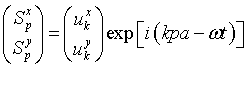Dispersion relation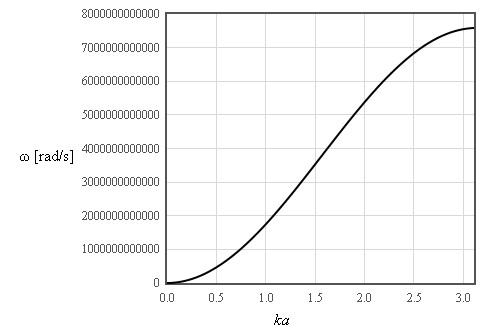Density of states D(ω)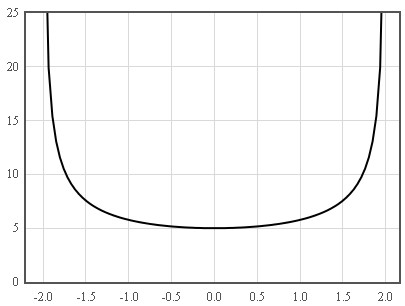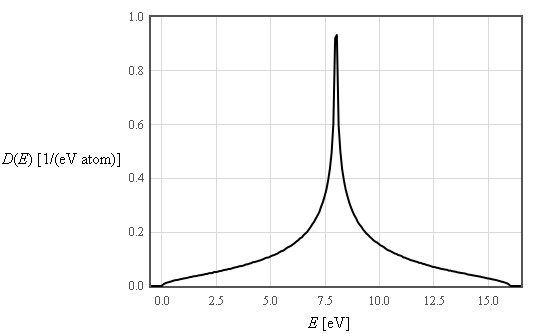Energy spectra1 density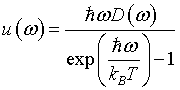Internal energy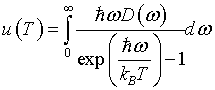Helmholtz free energyEntropy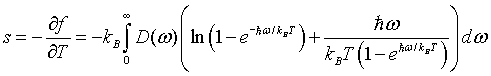Specific heat